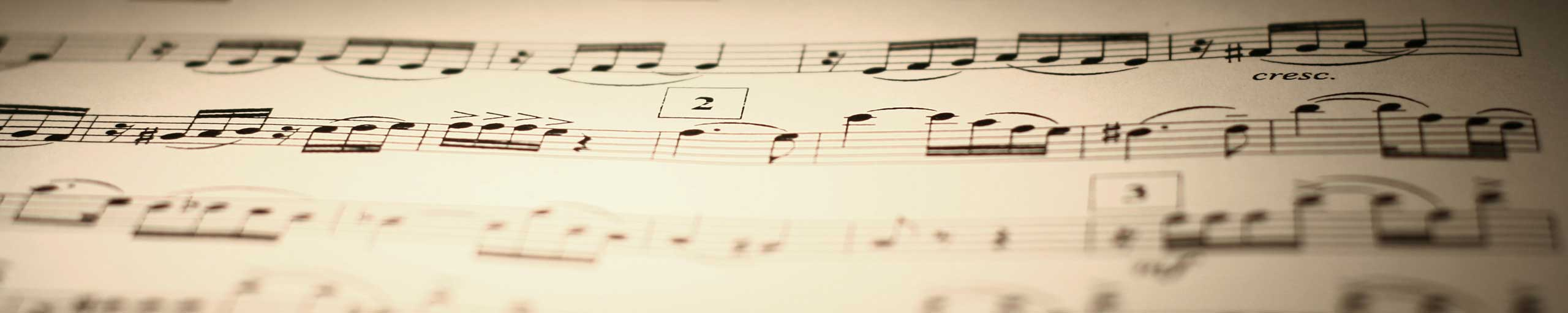# Convert Angles from Degrees, Minutes and Seconds to Decimal

An online calculator to convert angles from degrees, minutes and seconds to decimal using the table of conversion below.

## Table of Conversion

1 degree = 60 minutes
1 minute = 60 seconds.
1 degree = 3600 seconds
If an angle is given in the form: Degrees (�), minutes ('), seconds (") then the conversion to decimal degrees is given by
decimal degrees = Degrees + minutes/ 60 + seconds / 3600
Example: 45� 34' 53" = 45 + 34/60 + 53 / 3600 = 45.581389 degrees

## How to Use the Calculator

1 - Enter the size of your angle in degrees, minutes and seconds format and press "enter" and enter the number of decimal places desired. The answer is in degrees decimal format.
 Degrees Minutes Seconds 45 34 53 Decimal Places = 6 Degrees (decimal) =